图像处理 直线_图像处理,移除直线 - CSDN
• 图像处理之霍夫变换（直线检测算法） 霍夫变换是图像变换中的经典手段之一，主要用来从图像中分离出具有某种相同特征的几何 形状（如，直线，圆等）。霍夫变换寻找直线与圆的方法相比与其它方法可以更好的减少噪 声...
图像处理之霍夫变换（直线检测算法）霍夫变换是图像变换中的经典手段之一，主要用来从图像中分离出具有某种相同特征的几何形状（如，直线，圆等）。霍夫变换寻找直线与圆的方法相比与其它方法可以更好的减少噪声干扰。经典的霍夫变换常用来检测直线，圆，椭圆等。 霍夫变换算法思想：以直线检测为例，每个像素坐标点经过变换都变成都直线特质有贡献的统一度量，一个简单的例子如下：一条直线在图像中是一系列离散点的集合，通过一个直线的离散极坐标公式，可以表达出直线的离散点几何等式如下：X *cos(theta) + y * sin(theta)  = r 其中角度theta指r与X轴之间的夹角，r为到直线几何垂直距离。任何在直线上点，x, y都可以表达，其中 r， theta是常量。该公式图形表示如下：然而在实现的图像处理领域，图像的像素坐标P(x, y)是已知的，而r, theta则是我们要寻找的变量。如果我们能绘制每个(r, theta)值根据像素点坐标P(x, y)值的话，那么就从图像笛卡尔坐标系统转换到极坐标霍夫空间系统，这种从点到曲线的变换称为直线的霍夫变换。变换通过量化霍夫参数空间为有限个值间隔等分或者累加格子。当霍夫变换算法开始，每个像素坐标点P(x, y)被转换到(r, theta)的曲线点上面，累加到对应的格子数据点，当一个波峰出现时候，说明有直线存在。同样的原理，我们可以用来检测圆，只是对于圆的参数方程变为如下等式：(x –a ) ^2 + (y-b) ^ 2 = r^2其中(a, b)为圆的中心点坐标，r圆的半径。这样霍夫的参数空间就变成一个三维参数空间。给定圆半径转为二维霍夫参数空间，变换相对简单，也比较常用。 编程思路解析：1.      读取一幅带处理二值图像，最好背景为黑色。2.      取得源像素数据3.      根据直线的霍夫变换公式完成霍夫变换，预览霍夫空间结果4.       寻找最大霍夫值，设置阈值，反变换到图像RGB值空间(程序难点之一)5.      越界处理，显示霍夫变换处理以后的图像 关键代码解析：直线的变换角度为[0 ~ PI]之间，设置等份为500为PI/500，同时根据参数直线参数方程的取值范围为[-r, r]有如下霍夫参数定义： // prepare for hough transform
int centerX = width / 2;
int centerY = height / 2;
double hough_interval = PI_VALUE/(double)hough_space;

int max = Math.max(width, height);
int max_length = (int)(Math.sqrt(2.0D) * max);
hough_1d = new int[2 * hough_space * max_length];实现从像素RGB空间到霍夫空间变换的代码为：// start hough transform now....
int[][] image_2d = convert1Dto2D(inPixels);
for (int row = 0; row < height; row++) {
for (int col = 0; col < width; col++) {
int p = image_2d[row][col] & 0xff;
if(p == 0) continue; // which means background color

// since we does not know the theta angle and r value,
// we have to calculate all hough space for each pixel point
// then we got the max possible theta and r pair.
// r = x * cos(theta) + y * sin(theta)
for(int cell=0; cell < hough_space; cell++ ) {
max = (int)((col - centerX) * Math.cos(cell * hough_interval) + (row - centerY) * Math.sin(cell * hough_interval));
max += max_length; // start from zero, not (-max_length)
if (max < 0 || (max >= 2 * max_length)) {// make sure r did not out of scope[0, 2*max_lenght]
continue;
}
hough_2d[cell][max] +=1;
}
}
}寻找最大霍夫值计算霍夫阈值的代码如下：// find the max hough value
int max_hough = 0;
for(int i=0; i<hough_space; i++) {
for(int j=0; j<2*max_length; j++) {
hough_1d[(i + j * hough_space)] = hough_2d[i][j];
if(hough_2d[i][j] > max_hough) {
max_hough = hough_2d[i][j];
}
}
}
System.out.println("MAX HOUGH VALUE = " + max_hough);

// transfer back to image pixels space from hough parameter space
int hough_threshold = (int)(threshold * max_hough);从霍夫空间反变换回像素数据空间代码如下：	    // transfer back to image pixels space from hough parameter space
int hough_threshold = (int)(threshold * max_hough);
for(int row = 0; row < hough_space; row++) {
for(int col = 0; col < 2*max_length; col++) {
if(hough_2d[row][col] < hough_threshold) // discard it
continue;
int hough_value = hough_2d[row][col];
boolean isLine = true;
for(int i=-1; i<2; i++) {
for(int j=-1; j<2; j++) {
if(i != 0 || j != 0) {
int yf = row + i;
int xf = col + j;
if(xf < 0) continue;
if(xf < 2*max_length) {
if (yf < 0) {
yf += hough_space;
}
if (yf >= hough_space) {
yf -= hough_space;
}
if(hough_2d[yf][xf] <= hough_value) {
continue;
}
isLine = false;
break;
}
}
}
}
if(!isLine) continue;

// transform back to pixel data now...
double dy = Math.sin(row * hough_interval);
double dx = Math.cos(row * hough_interval);
if ((row <= hough_space / 4) || (row >= 3 * hough_space / 4)) {
for (int subrow = 0; subrow < height; ++subrow) {
int subcol = (int)((col - max_length - ((subrow - centerY) * dy)) / dx) + centerX;
if ((subcol < width) && (subcol >= 0)) {
image_2d[subrow][subcol] = -16776961;
}
}
} else {
for (int subcol = 0; subcol < width; ++subcol) {
int subrow = (int)((col - max_length - ((subcol - centerX) * dx)) / dy) + centerY;
if ((subrow < height) && (subrow >= 0)) {
image_2d[subrow][subcol] = -16776961;
}
}
}
}
}霍夫变换源图如下：霍夫变换以后，在霍夫空间显示如下：（白色表示已经找到直线信号）最终反变换回到像素空间效果如下：一个更好的运行监测直线的结果(输入为二值图像)：完整的霍夫变换源代码如下：package com.gloomyfish.image.transform;

import java.awt.image.BufferedImage;

import com.process.blur.study.AbstractBufferedImageOp;

public class HoughLineFilter extends AbstractBufferedImageOp {
public final static double PI_VALUE = Math.PI;
private int hough_space = 500;
private int[] hough_1d;
private int[][] hough_2d;
private int width;
private int height;

private float threshold;
private float scale;
private float offset;

public HoughLineFilter() {
// default hough transform parameters
//	scale = 1.0f;
//	offset = 0.0f;
threshold = 0.5f;
scale = 1.0f;
offset = 0.0f;
}

public void setHoughSpace(int space) {
this.hough_space = space;
}

public float getThreshold() {
return threshold;
}

public void setThreshold(float threshold) {
this.threshold = threshold;
}

public float getScale() {
return scale;
}

public void setScale(float scale) {
this.scale = scale;
}

public float getOffset() {
return offset;
}

public void setOffset(float offset) {
this.offset = offset;
}

@Override
public BufferedImage filter(BufferedImage src, BufferedImage dest) {
width = src.getWidth();
height = src.getHeight();

if ( dest == null )
dest = createCompatibleDestImage( src, null );

int[] inPixels = new int[width*height];
int[] outPixels = new int[width*height];
getRGB( src, 0, 0, width, height, inPixels );
houghTransform(inPixels, outPixels);
setRGB( dest, 0, 0, width, height, outPixels );
return dest;
}

private void houghTransform(int[] inPixels, int[] outPixels) {
// prepare for hough transform
int centerX = width / 2;
int centerY = height / 2;
double hough_interval = PI_VALUE/(double)hough_space;

int max = Math.max(width, height);
int max_length = (int)(Math.sqrt(2.0D) * max);
hough_1d = new int[2 * hough_space * max_length];

// define temp hough 2D array and initialize the hough 2D
hough_2d = new int[hough_space][2*max_length];
for(int i=0; i<hough_space; i++) {
for(int j=0; j<2*max_length; j++) {
hough_2d[i][j] = 0;
}
}

// start hough transform now....
int[][] image_2d = convert1Dto2D(inPixels);
for (int row = 0; row < height; row++) {
for (int col = 0; col < width; col++) {
int p = image_2d[row][col] & 0xff;
if(p == 0) continue; // which means background color

// since we does not know the theta angle and r value,
// we have to calculate all hough space for each pixel point
// then we got the max possible theta and r pair.
// r = x * cos(theta) + y * sin(theta)
for(int cell=0; cell < hough_space; cell++ ) {
max = (int)((col - centerX) * Math.cos(cell * hough_interval) + (row - centerY) * Math.sin(cell * hough_interval));
max += max_length; // start from zero, not (-max_length)
if (max < 0 || (max >= 2 * max_length)) {// make sure r did not out of scope[0, 2*max_lenght]
continue;
}
hough_2d[cell][max] +=1;
}
}
}

// find the max hough value
int max_hough = 0;
for(int i=0; i<hough_space; i++) {
for(int j=0; j<2*max_length; j++) {
hough_1d[(i + j * hough_space)] = hough_2d[i][j];
if(hough_2d[i][j] > max_hough) {
max_hough = hough_2d[i][j];
}
}
}
System.out.println("MAX HOUGH VALUE = " + max_hough);

// transfer back to image pixels space from hough parameter space
int hough_threshold = (int)(threshold * max_hough);
for(int row = 0; row < hough_space; row++) {
for(int col = 0; col < 2*max_length; col++) {
if(hough_2d[row][col] < hough_threshold) // discard it
continue;
int hough_value = hough_2d[row][col];
boolean isLine = true;
for(int i=-1; i<2; i++) {
for(int j=-1; j<2; j++) {
if(i != 0 || j != 0) {
int yf = row + i;
int xf = col + j;
if(xf < 0) continue;
if(xf < 2*max_length) {
if (yf < 0) {
yf += hough_space;
}
if (yf >= hough_space) {
yf -= hough_space;
}
if(hough_2d[yf][xf] <= hough_value) {
continue;
}
isLine = false;
break;
}
}
}
}
if(!isLine) continue;

// transform back to pixel data now...
double dy = Math.sin(row * hough_interval);
double dx = Math.cos(row * hough_interval);
if ((row <= hough_space / 4) || (row >= 3 * hough_space / 4)) {
for (int subrow = 0; subrow < height; ++subrow) {
int subcol = (int)((col - max_length - ((subrow - centerY) * dy)) / dx) + centerX;
if ((subcol < width) && (subcol >= 0)) {
image_2d[subrow][subcol] = -16776961;
}
}
} else {
for (int subcol = 0; subcol < width; ++subcol) {
int subrow = (int)((col - max_length - ((subcol - centerX) * dx)) / dy) + centerY;
if ((subrow < height) && (subrow >= 0)) {
image_2d[subrow][subcol] = -16776961;
}
}
}
}
}

// convert to hough 1D and return result
for (int i = 0; i < this.hough_1d.length; i++)
{
int value = clamp((int)(scale * this.hough_1d[i] + offset)); // scale always equals 1
this.hough_1d[i] = (0xFF000000 | value + (value << 16) + (value << 8));
}

// convert to image 1D and return
for (int row = 0; row < height; row++) {
for (int col = 0; col < width; col++) {
outPixels[(col + row * width)] = image_2d[row][col];
}
}
}

public BufferedImage getHoughImage() {
BufferedImage houghImage = new BufferedImage(hough_2d.length, hough_space, BufferedImage.TYPE_4BYTE_ABGR);
setRGB(houghImage, 0, 0, hough_2d.length, hough_space, hough_1d);
return houghImage;
}

public static int clamp(int value) {
if (value < 0)
value = 0;
else if (value > 255) {
value = 255;
}
return value;
}

private int[][] convert1Dto2D(int[] pixels) {
int[][] image_2d = new int[height][width];
int index = 0;
for(int row = 0; row < height; row++) {
for(int col = 0; col < width; col++) {
index = row * width + col;
image_2d[row][col] = pixels[index];
}
}
return image_2d;
}

}
转载文章请务必注明出自本博客！！学习图像处理，点击视频教程《数字图像处理-基础入门》
展开全文图像处理 算法 image float equals
• 本文主要整理自笔者在一项图像处理任务中的直线检测（line detection）部分的笔记资料，采用了基于霍夫变换（Hough Transform）的直线检测算法。文中给出了直线检测常用的算法介绍，论文资料等，以及笔者的实验笔记...
前言

[图像处理] 实验笔记系列是以图像处理算法为主的文章专栏，以我在算法研究中的实验笔记资料为基础加以整理推出的。该系列内容涉及常见的图像处理算法理论以及常见的算法应用，每篇博客都会介绍相关的算法原理，代码实现和算法在实际应用中的技巧。
本文主要整理自笔者在一项图像处理任务中的直线检测（line detection）部分的笔记资料，采用了基于霍夫变换（Hough Transform）的直线检测算法。文中给出了直线检测常用的算法介绍，论文资料等，以及笔者的实验笔记和实验结果。

文章小节安排如下：
1）直线检测相关算法
2）霍夫直线检测的基本原理
3）霍夫直线检测的OpenCV实现
4）直线检测的应用

一、直线检测相关算法

1.1 霍夫变换（Hough Transform）

霍夫变换（Hough Transform）换于1962年由Paul Hough 首次提出，后于1972年由Richard Duda和Peter Hart推广使用，是图像处理中从图像中检测几何形状的基本方法之一。经典霍夫变换用来检测图像中的直线，后来霍夫变换经过扩展可以进行任意形状物体的识别，例如圆和椭圆。

霍夫变换运用两个坐标空间之间的变换将在一个空间中具有相同形状的曲线或直线映射到另一个坐标空间的一个点上形成峰值，从而把检测任意形状的问题转化为统计峰值问题。

参考论文：
 P.V.C. Hough,Machine Analysis of Bubble Chamber Pictures, Proc. Int. Conf. High Energy Accelerators and Instrumentation, 1959.
 Duda, R. O. and P. E. Hart, “Use of the Hough Transformation to Detect Lines and Curves in Pictures,”Comm. ACM, Vol. 15, pp. 11–15 (January, 1972).

1.2 霍夫直线检测（Hough Line Detection）

Hough直线检测的基本原理在于利用点与线的对偶性，在我们的直线检测任务中，即图像空间中的直线与参数空间中的点是一一对应的，参数空间中的直线与图像空间中的点也是一一对应的。这意味着我们可以得出两个非常有用的结论：
1）图像空间中的每条直线在参数空间中都对应着单独一个点来表示；
2）图像空间中的直线上任何一部分线段在参数空间对应的是同一个点。

因此Hough直线检测算法就是把在图像空间中的直线检测问题转换到参数空间中对点的检测问题，通过在参数空间里寻找峰值来完成直线检测任务。

1.3 LSD

-待续-

二、霍夫直线检测的基本原理

2.1 关于对偶性

首先，我们通过实例来解释一下对偶性的意义。
1）图像空间中的点与参数空间中的直线一一对应
在图像空间x-y中一条直线在直角坐标系下可以表示为：其中k和b是参数，对应表示斜率和截距。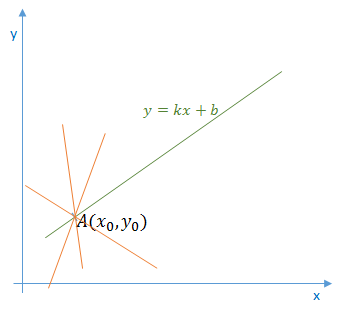过某一点A(x0, y0)的所有直线的参数均满足方程y0=k*x0+b，即点A(x0, y0)确定了一族直线。
如果我们将方程改写为：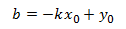那么该方程在参数空间k-b中就对应了一条直线：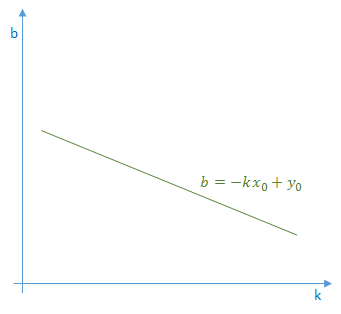也就是说，图像空间x-y中的点(x0,y0)对应了参数空间k-b中的直线b=-k*x0+y0。因此可以得到结论，图像空间中的点与参数空间中的直线一一对应。

2）图像空间中的直线与参数空间中的点一一对应
我们在直线y=k*x+b上再增加一个点B(x1, y1)，如下图所示：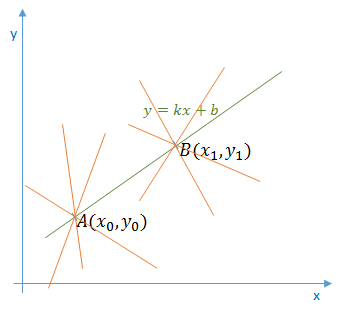那么点B(x1, y1)在参数空间同样对应了一条直线：可以看到，图像空间x-y中的点A和点B在参数空间k-b中对应的直线相交于一点，这也就是说AB所确定的直线，在参数空间中对应着唯一一个点，这个点的坐标值（k0, b0）也就是直线AB的参数。

以上就是在直线检测任务中关于对偶性的直观解释。这个性质也为我们解决直线检测任务提供了方法，也就是把图像空间中的直线对应到参数空间中的点，最后通过统计特性来解决问题。假如图像空间中有两条直线，那么最终在参数空间中就会对应到两个峰值点，依此类推。

2.2 参数空间的选择

上述为了方便讲解对偶性和霍夫变换的基本原理，我们的参数空间也选择了笛卡尔直角坐标系。但在实际应用中，参数空间是不能选择直角坐标系的，因为原始图像直角坐标空间中的特殊直线x=c（垂直x轴，直线的斜率为无穷大）是没办法在基于直角坐标系的参数空间中表示的。

所以在实际应用中，参数空间采用极坐标系ρ-θ，图示如下：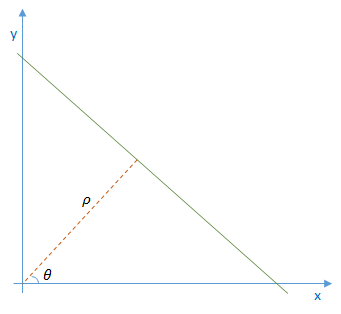直线的表达式为：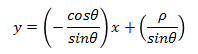化简便可得到：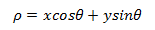对于直线上的点(x0, y0)，可以将通过该点的直线族定义为：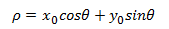这就回到我们刚才的结论，参数空间的每个点(ρ,θ)都对应了图像空间的一条直线，或者说图像空间的一个点在参数空间中就对应为一条曲线。参数空间采用极坐标系，这样就可以在参数空间表示原始空间中的所有直线了。
注意，此时图像空间（直角坐标系x-y）上的一个点对应到参数空间（极坐标系ρ-θ）上是一条曲线，确切的说是一条正弦曲线。2.3 利用霍夫变换检测直线

如前所述，霍夫直线检测就是把图像空间中的直线变换到参数空间中的点，通过统计特性来解决检测问题。具体来说，如果一幅图像中的像素构成一条直线，那么这些像素坐标值（x, y）在参数空间对应的曲线一定相交于一个点，所以我们只需要将图像中的所有像素点（坐标值）变换成参数空间的曲线，并在参数空间检测曲线交点就可以确定直线了。

在理论上，一个点对应无数条直线或者说任意方向的直线，但在实际应用中，我们必须限定直线的数量（即有限数量的方向）才能够进行计算。

因此，我们将直线的方向θ离散化为有限个等间距的离散值，参数ρ也就对应离散化为有限个值，于是参数空间不再是连续的，而是被离散量化为一个个等大小网格单元。将图像空间（直角坐标系）中每个像素点坐标值变换到参数空间（极坐标系）后，所得值会落在某个网格内，使该网格单元的累加计数器加1。当图像空间中所有的像素都经过霍夫变换后，对网格单元进行检查，累加计数值最大的网格，其坐标值（ρ0, θ0）就对应图像空间中所求的直线。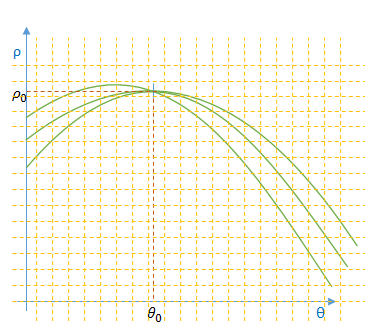以上就是霍夫直线检测算法要做的，它检测图像中每个像素点在参数空间对应曲线之间的交点，如果交于一点的曲线的数量超过了阈值，那就可以认为这个交点(ρ,θ)在图像空间中对应一条直线。

2.4 霍夫直线检测的优缺点

优点：
Hough直线检测的优点是抗干扰能力强，对图像中直线的殘缺部分、噪声以及其它共存的非直线结构不敏感。
缺点：
Hough变换算法的特点导致其时间复杂度和空间复杂度都很高，并且在检测过程中只能确定直线方向，丢失了线段的长度信息。

三、霍夫直线检测的OpenCV实现

OpenCV支持三种霍夫直线检测算法：
1）Standard Hough Transform(SHT，标准霍夫变换)
2）Multiscale Hough Transform(MSHT，多尺度霍夫变换)
3）Progressive Probability Houth Transform(PPHT，渐进概率式霍夫变换)

3.1 霍夫直线检测函数定义

在OpenCV2.1之前的版本，霍夫直线检测函数如下：

函数原型：

CVAPI(CvSeq*)  cvHoughLines2( CvArr* image, void* line_storage, int method,
double rho, double theta, int threshold,
double param1 CV_DEFAULT(0), double param2 CV_DEFAULT(0),
double min_theta CV_DEFAULT(0), double max_theta CV_DEFAULT(CV_PI));

函数说明：
cvHoughLines2老版OpenCV的霍夫直线检测函数，通过method参数可以支持三种霍夫直线检测算法，分别是CV_HOUGH_STANDARD、CV_HOUGH_PROBABILISTIC =1、CV_HOUGH_MULTI_SCALE。

在OpenCV新版本下，霍夫直线检测算法定义了两个函数：HoughLines、HoughLinesP
1）HoughLines：标准霍夫变换、多尺度霍夫变换
函数原型：

CV_EXPORTS_W void HoughLines( InputArray image, OutputArray lines,
double rho, double theta, int threshold,
double srn = 0, double stn = 0,
double min_theta = 0, double max_theta = CV_PI );

参数说明：
InputArray image：输入图像，必须是8位单通道图像。
OutputArray lines：检测到的线条参数集合。
double rho：以像素为单位的距离步长。
double theta：以弧度为单位的角度步长。
int threshold：累加计数值的阈值参数，当参数空间某个交点的累加计数的值超过该阈值，则认为该交点对应了图像空间的一条直线。
double srn：默认值为0，用于在多尺度霍夫变换中作为参数rho的除数，rho=rho/srn。
double stn：默认值为0，用于在多尺度霍夫变换中作为参数theta的除数，theta=theta/stn。

函数说明：
HoughLines函数输出检测到直线的矢量表示集合，每一条直线由具有两个元素的矢量（ρ, θ）表示，其中ρ表示直线距离原点（0, 0）的长度，θ表示直线的角度（以弧度为单位）。
HoughLines函数无法输出图像空间中线段的长度，这也是霍夫变换本身的弱点。

备注说明：
如果srn和stn同时为0，就表示HoughLines函数执行标准霍夫变换，否则就是执行多尺度霍夫变换。

2）HoughLinesP：渐进概率式霍夫变换
函数原型：

CV_EXPORTS_W void HoughLinesP( InputArray image, OutputArray lines,
double rho, double theta, int threshold,
double minLineLength = 0, double maxLineGap = 0 );

参数说明：
InputArray image：输入图像，必须是8位单通道图像。
OutputArray lines：检测到的线条参数集合。
double rho：直线搜索时的距离步长，以像素为单位。
double theta：直线搜索时的角度步长，以弧度为单位。
int threshold：累加计数值的阈值参数，当参数空间某个交点的累加计数的值超过该阈值，则认为该交点对应了图像空间的一条直线。
double minLineLength：默认值为0，表示最小线段长度阈值（像素）。
double maxLineGap：默认值为0，表示直线断裂的最大间隔距离阈值。即如果有两条线段是在一条直线上，但它们之间有间隙，那么如果这个间隔距离大于该值，则被认为是一条线段，否则认为是两条线段。

函数说明：
HoughLinesP函数输出检测到直线的矢量表示集合，每一条直线由具有四个元素的矢量（x1, y1, x2, y2）表示，其中(x1, y1)表示线段的起点，(x2, y2)表示线段的终点。
HoughLinesP函数可以检测出图像空间中线段的长度。

3.2 霍夫直线检测函数使用

霍夫直线变换是一种用来在图像空间寻找直线的方法，输入图像要求是二值图像，同时为了提高检测直线的效率和准确率，在使用霍夫线变换之前，最好对图像进行边缘检测生成边缘二值图像，这样的检测效果是最好的。

1）HoughLines函数
代码：

std::string img_path;
cv::Mat mat_color;
cv::Mat mat_gray;
cv::Mat mat_binary;
cv::Mat mat_canny;
cv::Mat mat_board;

img_path = "line.png";
mat_board = cv::Mat(mat_color.size(), mat_color.type(), Scalar::all(255));

// binary
cv::threshold(mat_gray, mat_binary, 0.0, 255.0, cv::THRESH_OTSU);
// invert color
cv::bitwise_not(mat_binary, mat_binary);

// detect edge
Canny(mat_binary, mat_canny, 50, 200, 3);

// detect line
vector<Vec2f> lines;
HoughLines(mat_canny, lines, 1, CV_PI / 180, 150, 0, 0);

// draw line
cout << "line number: " << lines.size() << endl;
for (size_t i = 0; i < lines.size(); i++)
{
Vec2f linex = lines[i];
cout << "radius: " << linex << ", radian: "<< linex << ", angle: " << 180 / CV_PI * linex << endl;
float rho = lines[i], theta = lines[i];
Point pt1, pt2;
double a = cos(theta), b = sin(theta);
double x0 = a * rho, y0 = b * rho;
pt1.x = cvRound(x0 + 1000 * (-b));
pt1.y = cvRound(y0 + 1000 * (a));
pt2.x = cvRound(x0 - 1000 * (-b));
pt2.y = cvRound(y0 - 1000 * (a));
line(mat_board, pt1, pt2, Scalar(255, 0, 0), 1);
}
cv::imshow("gray", mat_gray);
cv::imshow("binary", mat_binary);
cv::imshow("canny", mat_canny);
cv::imshow("color", mat_board);
cv::waitKey();

原图：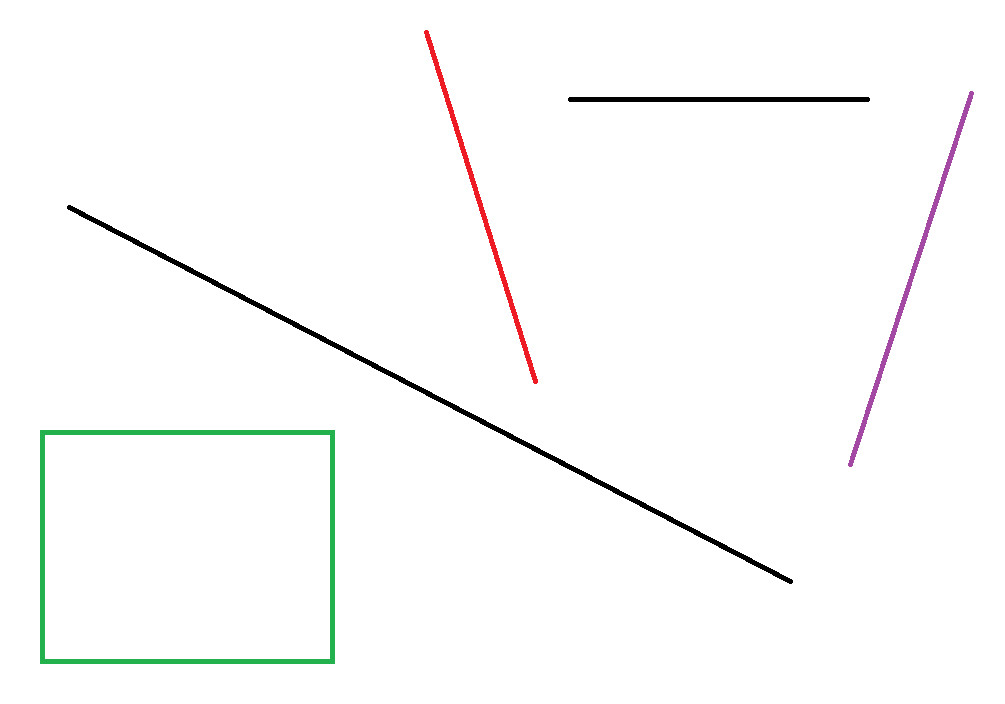二值图：边缘图：检测效果：2）HoughLinesP函数
代码：

std::string img_path;
cv::Mat mat_color;
cv::Mat mat_gray;
cv::Mat mat_binary;
cv::Mat mat_canny;
cv::Mat mat_board;

img_path = "line.png";
mat_board = cv::Mat(mat_color.size(), mat_color.type(), Scalar::all(255));

// binary
cv::threshold(mat_gray, mat_binary, 0.0, 255.0, cv::THRESH_OTSU);
// invert color
cv::bitwise_not(mat_binary, mat_binary);

// detect edge
Canny(mat_binary, mat_canny, 50, 200, 3);

// detect line
vector<Vec4i> lines;
HoughLinesP(mat_canny, lines, 1, CV_PI / 180, 150, 50, 50);

// draw line
cout << "line number: " << lines.size() << endl;
for (size_t i = 0; i < lines.size(); i++)
{
Vec4i linex = lines[i];
line(mat_board, Point(linex, linex), Point(linex, linex), Scalar(255, 0, 0), 1);
}

cv::imshow("gray",   mat_gray);
cv::imshow("binary", mat_binary);
cv::imshow("canny", mat_canny);
cv::imshow("color", mat_board);
cv::waitKey();

检测效果：四、直线检测的应用

4.1 直线检测的实际应用

直线检测是机器视觉和模式识别中最重要的任务之一，对图像理解/分析等有重要的意义。在实际应用中，直线检测可用于机器人定位中的网格识别，板材的裂纹检测，表单票据的格式识别，零件纹路的检测，自动驾驶中的车道检测等等，可以看出，在工业领域中，直线检测以及各种图像处理技术应用是非常丰富的。

4.2 直线检测在图像矫正方面的应用

笔者最近在一个OCR项目也使用了Hough Line Detection算法。在该项目中，待识别文本图像的内容是倾斜的（即文字是倾斜的），我们采用的测略就是通过直线检测确定图像内容的倾斜程度并进行旋转纠正，这样得到的无倾斜图像更有利于OCR任务。
原图：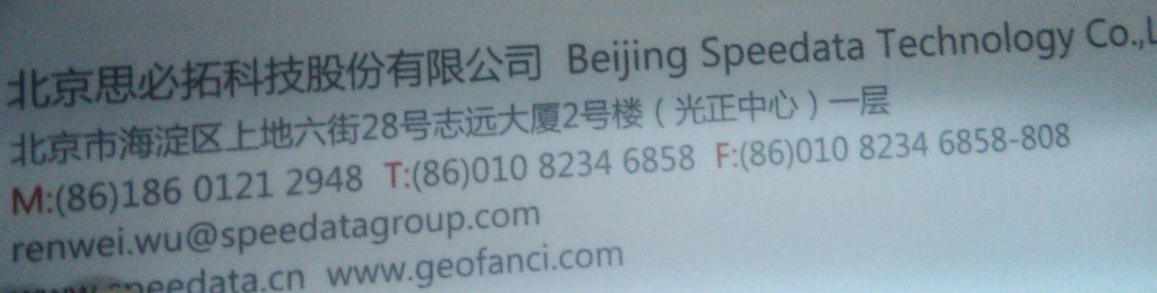矫正效果：五、参考资料

参考论文与书目：
 Duda, R. O. and P. E. Hart, “Use of the Hough Transformation to Detect Lines and Curves in Pictures,”Comm. ACM, Vol. 15, pp. 11–15 (January, 1972).
 顾思妍. 机器视觉的直线检测技术及应用研究[D].广东工业大学,2011.
 数字图像处理[M]. 电子工业出版社 , (美)RafaelC.Gonzalez,(美)RichardE.Woods,(美)StevenL.Eddins著, 2005

参考博客：
Hough变换-理解篇 http://blog.csdn.net/abcjennifer/article/details/7448513
Hough transform(霍夫变换) http://www.cnblogs.com/AndyJee/p/3805594.html
霍夫变换概述和标准霍夫变换 http://www.jianshu.com/p/55eabb42c6c2
展开全文图像处理 算法 霍夫变换
• 1.霍夫变换(Hough Transform) 霍夫变换是图像处理中从图像中识别几何形状的基本方法之一，应用很广泛，也有很多改进算法。主要用来从图像中分离出具有某种相同特征的几何形状（如，直线，圆等）。最基本的霍夫变换是...
简介：

1.霍夫变换(Hough Transform) 霍夫变换是图像处理中从图像中识别几何形状的基本方法之一，应用很广泛，也有很多改进算法。主要用来从图像中分离出具有某种相同特征的几何形状（如，直线，圆等）。最基本的霍夫变换是从黑白图像中检测直线(线段)。

2.Hough变换的原理是将特定图形上的点变换到一组参数空间上，根据参数空间点的累计结果找到一个极大值对应的解，那么这个解就对应着要寻找的几何形状的参数（比如说直线，那么就会得到直线的斜率k与常熟b，圆就会得到圆心与半径等等）

3.霍夫线变换是一种用来寻找直线的方法。用霍夫线变换之前, 首先需要对图像进行边缘检测的处理，也即霍夫线变换的直接输入只能是边缘二值图像。

4.霍夫直线检测的具体原理参见：

https://blog.csdn.net/ycj9090900/article/details/52944708

http://www.opencv.org.cn/opencvdoc/2.3.2/html/doc/tutorials/imgproc/imgtrans/hough_lines/hough_lines.html

http://lib.csdn.net/article/opencv/24201

代码如下：#直线检测
#使用霍夫直线变换做直线检测，前提条件：边缘检测已经完成
import cv2 as cv
import numpy as np

#标准霍夫线变换
def line_detection(image):
gray = cv.cvtColor(image, cv.COLOR_RGB2GRAY)
edges = cv.Canny(gray, 50, 150, apertureSize=3)  #apertureSize参数默认其实就是3
cv.imshow("edges", edges)
lines = cv.HoughLines(edges, 1, np.pi/180, 80)
for line in lines:
rho, theta = line  #line存储的是点到直线的极径和极角，其中极角是弧度表示的。
a = np.cos(theta)   #theta是弧度
b = np.sin(theta)
x0 = a * rho    #代表x = r * cos（theta）
y0 = b * rho    #代表y = r * sin（theta）
x1 = int(x0 + 1000 * (-b)) #计算直线起点横坐标
y1 = int(y0 + 1000 * a)    #计算起始起点纵坐标
x2 = int(x0 - 1000 * (-b)) #计算直线终点横坐标
y2 = int(y0 - 1000 * a)    #计算直线终点纵坐标    注：这里的数值1000给出了画出的线段长度范围大小，数值越小，画出的线段越短，数值越大，画出的线段越长
cv.line(image, (x1, y1), (x2, y2), (0, 0, 255), 2)    #点的坐标必须是元组，不能是列表。
cv.imshow("image-lines", image)

#统计概率霍夫线变换
def line_detect_possible_demo(image):
gray = cv.cvtColor(image, cv.COLOR_RGB2GRAY)
edges = cv.Canny(gray, 50, 150, apertureSize=3)  # apertureSize参数默认其实就是3
lines = cv.HoughLinesP(edges, 1, np.pi / 180, 60, minLineLength=60, maxLineGap=5)
for line in lines:
x1, y1, x2, y2 = line
cv.line(image, (x1, y1), (x2, y2), (0, 0, 255), 2)
cv.imshow("line_detect_possible_demo",image)

print(src.shape)
cv.namedWindow('input_image', cv.WINDOW_AUTOSIZE)
cv.imshow('input_image', src)
line_detection(src)
line_detect_possible_demo(src)
cv.waitKey(0)
cv.destroyAllWindows()运行结果：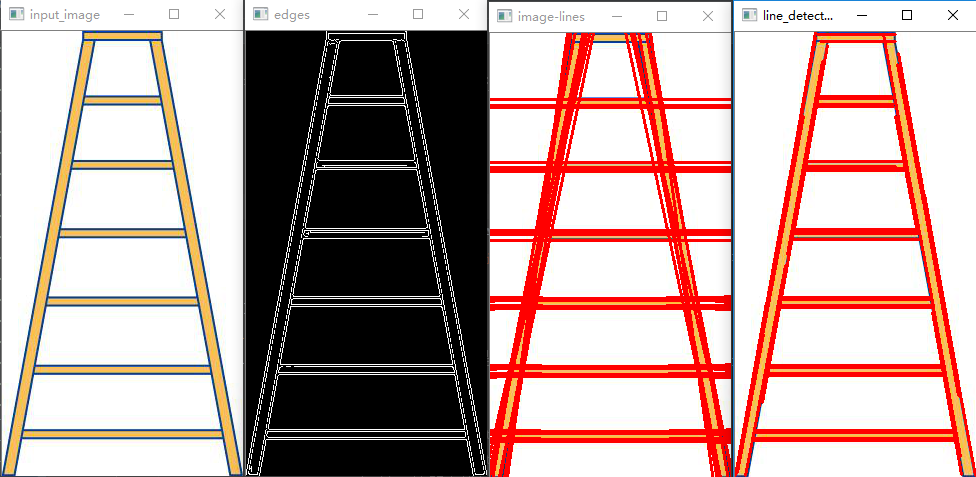注意：

1.opencv的HoughLines函数是标准霍夫线变换函数，该函数的功能是通过一组参数对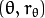的集合来表示检测到的直线，其函数原型为：HoughLines(image, rho, theta, threshold[, lines[, srn[, stn[, min_theta[, max_theta]]]]]) -> lines

image参数表示边缘检测的输出图像，该图像为单通道8位二进制图像。

rho参数表示参数极径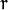以像素值为单位的分辨率，这里一般使用1像素。

theta参数表示参数极角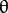以弧度为单位的分辨率，这里使用1度。

threshold参数表示检测一条直线所需最少的曲线交点。

lines参数表示储存着检测到的直线的参数对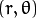的容器 。

srn参数、stn参数默认都为0。如果srn = 0且stn = 0，则使用经典的Hough变换。

min_theta参数表示对于标准和多尺度Hough变换，检查线条的最小角度。

max_theta参数表示对于标准和多尺度Hough变换，检查线条的最大角度。

2.opencv的HoughLinesP函数是统计概率霍夫线变换函数，该函数能输出检测到的直线的端点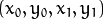，其函数原型为：HoughLinesP(image, rho, theta, threshold[, lines[, minLineLength[, maxLineGap]]]) -> lines

image参数表示边缘检测的输出图像，该图像为单通道8位二进制图像。

rho参数表示参数极径以像素值为单位的分辨率，这里一般使用 1 像素。

theta参数表示参数极角以弧度为单位的分辨率，这里使用 1度。

threshold参数表示检测一条直线所需最少的曲线交点。

lines参数表示储存着检测到的直线的参数对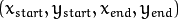的容器，也就是线段两个端点的坐标。

minLineLength参数表示能组成一条直线的最少点的数量，点数量不足的直线将被抛弃。

maxLineGap参数表示能被认为在一条直线上的亮点的最大距离
展开全文• 霍夫变换是图像处理中从图像中识别几何形状的基本方法之一，应用很广泛，也有很多改进算法。主要用来从图像中分离出具有某种相同特征的几何形状（如，直线，圆等）。 python实现 import cv2 import numpy as np...
霍夫变换是图像处理中从图像中识别几何形状的基本方法之一，应用很广泛，也有很多改进算法。主要用来从图像中分离出具有某种相同特征的几何形状（如，直线，圆等）。
python实现

import cv2
import numpy as np
# 使用霍夫直线变换做直线检测，前提条件：边缘检测已经完成

__author__ = "boboa"

# 标准霍夫线变换
def line_detection_demo(image):
gray = cv2.cvtColor(image, cv2.COLOR_BGR2GRAY)
edges = cv2.Canny(gray, 50, 150, apertureSize=3)
lines = cv2.HoughLines(edges, 1, np.pi/180, 200)  # 函数将通过步长为1的半径和步长为π/180的角来搜索所有可能的直线
for line in lines:
rho, theta = line  # line存储的是点到直线的极径和极角，其中极角是弧度表示的
a = np.cos(theta)   # theta是弧度
b = np.sin(theta)
x0 = a * rho
y0 = b * rho
x1 = int(x0 + 1000 * (-b))  # 直线起点横坐标
y1 = int(y0 + 1000 * (a))   # 直线起点纵坐标
x2 = int(x0 - 1000 * (-b))  # 直线终点横坐标
y2 = int(y0 - 1000 * (a))   # 直线终点纵坐标
cv2.line(image, (x1, y1), (x2, y2), (0, 0, 255), 2)
cv2.imshow("image_lines", image)

# 统计概率霍夫线变换
def line_detect_possible_demo(image):
gray = cv2.cvtColor(image, cv2.COLOR_BGRA2GRAY)
edges = cv2.Canny(gray, 50, 150, apertureSize=3)
# 函数将通过步长为1的半径和步长为π/180的角来搜索所有可能的直线
lines = cv2.HoughLinesP(edges, 1, np.pi / 180, 100, minLineLength=50, maxLineGap=10)
for line in lines:
x1, y1, x2, y2 = line
cv2.line(image, (x1, y1), (x2, y2), (0, 0, 255), 2)
cv2.imshow("line_detect_possible_demo", image)

if __name__ == "__main__":
cv2.namedWindow("input image", cv2.WINDOW_AUTOSIZE)
cv2.imshow("input image", img)
line_detect_possible_demo(img)
cv2.waitKey(0)
cv2.destroyAllWindows()

标准霍夫线变换运行结果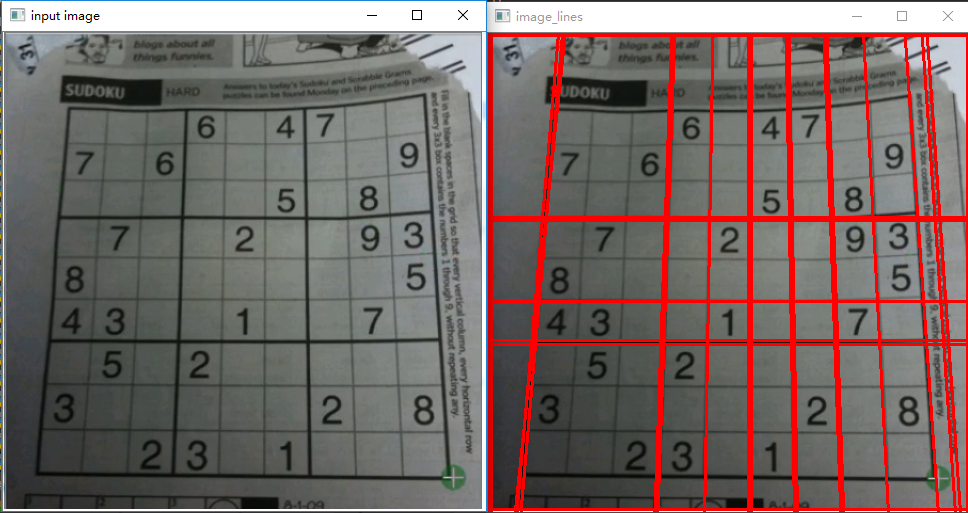统计概率霍夫线变换运行结果标准霍夫线变换cv2.HoughLines(image, rho, theta, threshold[, lines[, srn[, stn[, min_theta[, max_theta]]]]]) -> lines
参数：image-边缘检测的输出图像，8位，单通道二进制源图像
rho-距离步长
theta-角度步长
threshold-阈值，只有大于该值的点才有可能被当作极大值，即至少有多少条正弦曲线交于一点才被认为是直线

统计概率霍夫线变换cv2.HoughLinesP(image, rho, theta, threshold[, lines[, minLineLength[, maxLineGap]]]) -> lines
参数：image-边缘检测的输出图像，该图像为单通道8位二进制图像
rho-参数极径以像素值为单位的分辨率，这里一般使用 1 像素
theta-参数极角以弧度为单位的分辨率，这里使用 1度
threshold-检测一条直线所需最少的曲线交点
minLineLength-线的最短长度，比这个线短的都会被忽略
maxLineGap-两条线之间的最大间隔，如果小于此值，这两条线就会被看成一条线。
HoughLinesP，效果更好，检测图像中分段的直线（而不是贯穿整个图像的直线）

转载于:https://www.cnblogs.com/qianxia/p/11101758.html
展开全文• 1.最小二乘拟合 最简单，但是受离群点的影响比较大，鲁棒性不强。2.梯度下降法3.高斯牛顿、列-马算法直线拟合
• P.V.C.Hough第一次提出用霍夫变换（Hough Transform） 检测二值图中的直线和曲线， R.D.Duda和P.E.Hart根据霍夫变换提出了一种更有效的直线检测方法， 这就是通常所称的标准霍夫直线检测。 下面详细介绍它的...
• 我的图像处理的一个作业。 1.任务 PCB图的直线提取 2.使用平台 Windows10专业版 VS2015企业版 C++ opencv3.2 3.图像处理的思路 第一部分：图像的前期处理。 二值化、滤波、形态学操作等等 第二部分：直线检测与标记...图像处理 形态学操作
• 边缘连接 我们得到边缘图以后会出现是一个问题，就是由于有的像素偏暗，会出现边缘不连续的现象，这种情况我们就要采用边缘连接算法。我们对每一个边缘上的点进行遍历，以这个像素为中心看一个矩形窗内的其他像素，...数字图像处理 多边形拟合c
• 本文转自：... 例如：像素的取值范围为0-255，就称该图像为256个灰度级的图像 2）层次：表示图像实际拥有的灰度级的数量 例如：具有32种不同取值的图像，可称该图像具有32个层次 图图像处理
• ## 图像处理基础

千次阅读 2014-07-27 10:29:59
眼睛是心灵的窗口，至少有80%以上的外界信息经视觉获得，视觉是人和动物最重要的感觉。java 图像处理
• 遥感图像的机场检测是图像处理在军事以及航空领域一个重要的应用，现有一些机场提取方法利用显著性特征获取机场区域的方法容易使得机场提取不够完整，而且会混入过多的虚警区域，原因在于图像的显著性特征并能用来...
•图像处理
• GIMP - GNU 图像处理程序 - 画直线 1. Pencil Tool & Size 2. 在直线的起点单击，按住 shift 键，然后点击直线的终点位置。
• 前言 Opencv4图像分割和识别实战6的视频课程...1）通过前面所学的投影分割法来分别获得这2条直线上的离散采样点，然后将它们通过直线拟合求得各自的直线方程，如k1x+b和k2x+b2。...连通域分析
• ## 检测 图像中得直线

千次阅读 2018-11-10 12:52:56